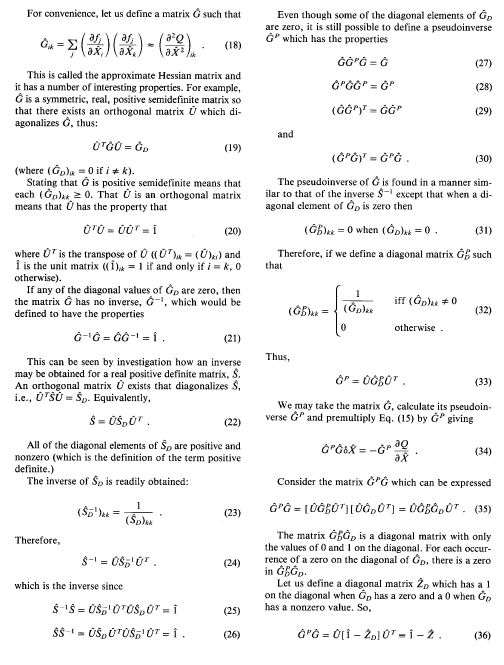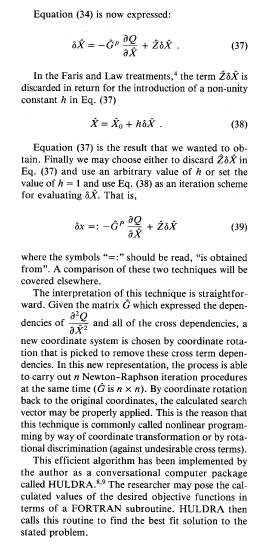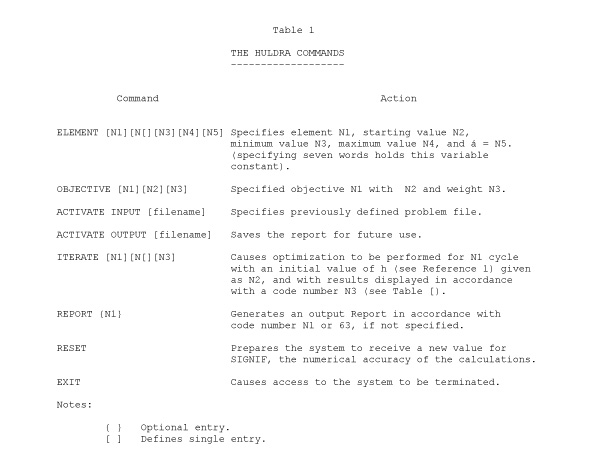# HULDRA, the SCI Non-Linear Regression (Optimization) Package

HULDRA is a library of subroutines, callable from Fortran, and C, to carry out rotational- discrimination non-linear regression analysis (optimization.) This library of subroutines was written by John H. Letcher Professor of Computer Science of the University of Tulsa and President of Synergistic Consultants Incorporated,

In Teutonic mythology, Huldra is the Goddess who is always aspiring to the unattainable. SCI felt that this name was appropos for this tool.

HULDRA is a nonlinear optimizer enabling the user to determine an optimum numerical solution to a problem. The user must quantitatively specify the variables to be considered in the solution of the problem, the goals or objectives that he wishes to achieve and the values of functions defined in terms of the variables (objectives). The functional relationships between the variables and the objectives must be defined. These are designated to the system as ELEMENTS, OBJECTIVES, and the VALUE subroutine, respectively. The system calculates the values of the elements to produce the best fit to the specified objectives. The parameters to drive the calculation are given as a set of concise commands.

The functional relationships are expressed by way of a FORTRAN-77 or C subroutine, called VALUE. These may be as complicated as is required to state the problem. The restriction must be obeyed that each objective must be differentiable (in the mathematical sense); other than that single restriction the evaluation may be extraordinarily complex even including calls to data base access subroutines for supporting information.

The act of performing the optimization is an affair independent of the statement of the problem as the choice of goals and the degree of constraint on the elements is not known at the time of the statement of the problem. Therefore, to use a previously defined problem, the user need not know FORTRAN or any computer language. The terse HULDRA operating commands are easily understood and used.

HULDRA operates in an interactive (conversational) mode as well as in the batch mode so that planners can test different assumptions and approaches and obtain results in real time.

General system features are:

1. The functional relationships between the elements and objectives may be nonlinear. This removes a primary restriction of linear programming systems and permits consideration of "real world" problems.

2. The chosen elements need not be linearly independent. In complicated problems, it is seldom known at the onset the relationships, if any, between the "independent variables" (elements).

3. The calculational procedure is independent of the elements upon which optimization is to be performed. For example, the system could be used for the optimization of land use and allocation of money in separate programs with a common language and a common output format to preclude any confusion in the interpretation of the results of the optimization procedure.

4. The system user is able to define bounds on the magnitude of the value of an element irrespective of the magnitude that would have been calculated in an unconstrained optimization.

5. Relative weights are given to each objective to give priorities that the calculational procedure obeys in case all of the defined objectives cannot be realized.

6. HULDRA will not diverge; that is, it is impossible to formulate a problem that will cause the final values to be further from the specified objectives than their starting points.

7. The size of the problems considered is limited primarily by the computer memory available. For a modern microcomputer, such as a PC, normally, a problem with 20 elements and 10 objectives can be specified easily. But, problems of virtually unlimited size may be specified. The solution algorithm is efficient in terms of calculation time.

8. The HULDRA System approaches a solution in steps. That is, after one step (called an iteration) the values of all of the elements may change and the calculated values of the objective functions are closer to the desired values than in the previous step. That is, HULDRA is said to be an iterative process. The results at each step may be meaningful, per se. Iteration to completion need not be accomplished to give useful information to the user.

The following sections discuss the mathematic formulation of the problem within the system, problem specification to the system, system commands, error messages, and a sample problem illustrating the techniques of system utilization.

## 1. Mathematical Formulation

The planning system is a solution technique for a problem well known in the mathematical literature. The Appendix in this reference offers a concise definition of the process. Also, this reference give a more detailed description of the mathematical basis for Huldra. A summary of the algorithm is given, below:So, in the Huldra System which is described in this document, in terms of a set of elements, X(I), which is the same as Xi, and a set of objective functions F(J), the total objective function, Q, can be calculated with good accuracy in terms of the values of the elements, X(I). The purpose of the calculational procedure is to minimize the sum of the squares of the differences between the calculated value of these objective functions, F(J) and the defined goals, G(J), multiplied by the values of the weights, W(J).

The weights, W(J), offer relative importance factors to each of the defined objectives. An element is an entity characterized by a set of attributes: its value is X(I); the maximum value that this entity should be allowed to attain is XMAX(I); the minimum value that the quantity should attain is XMIN(I).

The Huldra algorithm for carrying out this minimization is extremely efficient; however, if used directly, it embodies all of the problems associated with numerical differentiation of functions on a digital computer.

Sensitivity coefficients can be defined which give to the system user a figure of merit of the anticipated change that one would expect in the calculated value of a single objective function in terms of minor variations of the calculated value of each X. Mathematically, these are the partial derivatives of F with respect to X, and are shown as a part of the derivation shown, above.

Two of the attributes associated with each element are the maximum and minimum values that each element can attain within the course of the calculation. This is true rigorously where indeed the value may not exceed the bounds of these quantities to any extent (hard boundary conditions).

## 2. Problem Specification

The specificaton of any problem for HULDRA requires only three types of input parameters: ELEMENT, OBJECTIVE, and Objective function specification. It is in the specification of these parameters that the planner's knowledge of his particular problem is implemented. He must know what his real goals are, what techniques are to be considered to achieve these goals, and, perhaps most importantly, the approximate relationship between the techniques and the goal. To reiterate, the HULDRA System is designed to find the optimum combination of many alternative techniques to achieve particular goals. However, the basic relationship between the technique and the goal is the responsibility of the planner. This relationship should be understood to solve the problem in any manner, but use of the HULDRA system forces the planner to quantitatively define the interaction. Since the relationships are easily changed and the computer is very fast, a variety of assumptions can be tested in a few minutes once the basic problem has been defined to the machine. In this manner the degree of criticalness of the assumptions can be determined and primary attention given to verifying the validity of the dominant ones.

### 2.1 ELEMENT

Each problem is formulated as a set of independent variables called elements.

In addition to its arbitrarily chosen element number, the starting value as well as the maximum and minimum permissible values for each element must be specified to the system. In complicated problems there is the possibility of multiple peaks with different values for the objectives. To insure that such a situation does not exist, it is "good form" to start the solution at several different points and verify that identical results are obtained.

Specification of maximum and minimum element values keeps the results obtained physically realizable.

With the HULDRA programs the values of the objective functions, F(J) are evaluated. These objective functions are functions of the X(J) values. Furthermore, the partial derivative of each objective function with respect to each independent variable X uses the method of central differences. This uses a small increment, E(I)

The increment, Ei must be explicitly specified for each Xi as the sixth word in the ELEMENT Command which is described later. The above states that the technique of central differences is used in the evaluation of all partial derivatives within HULDRA.

An optional specification to the HULDRA system is for particular elements to be held constant. This can be done for any element, simply by specifying a sixth word in the ELEMENT command.

### 2.2 OBJECTIVE

Specification of the objectives to the system defines the goals of the planning process. Three quantities must be specified for each objective - a number, a value which is the "goal", and a relative importance factor called its weight. These are discussed in the following paragraphs.

The number is assigned for bookkeeping purposes, just as was done for each element. There are no conventions or rules for the numbering sequence.

A particular element can have multiple objective values associated with it.

Specification of a weight for each objective allows the planner to place a judgement on its relative importance. However, this is not as straightforward as it would at first appear. As is shown in Appendix B, the HULDRA calculational procedure sums the products of the weight and the square of the difference between the goal and the current value for each of the objectives. The purpose of the HULDRA system is to minimize the value of this total objective function, which is an inherently positive number. Obviously, if the goals are perfectly achieved, the quantity becomes zero. The reason selection of the weight may not be so obvious is that the total objective function contains the product of the weight and the square of the difference from each of the goals. If the size of the objectives varies greatly, minimizing the total objective function favors the objectives with the largest values. Thus, assigning each objective a weight of unity may in actuality result in a large discrepancy in the relative importance of each goal. This is particularly important when the goals cannot all be achieved. However, by varying the relative weights of the objectives, achievement of a particular goal can be easily emphasized.

## 3. Commands

The commands to manipulate the HULDRA System are summarized in Table 1 and are discussed below.

### 3.1 ELEMENT

This command is used to specify the element paramters to the system. The entries after the word ELEMENT are in free field format, i.e. they may be separated by spaces or commas. They may be expressed in fixed, floating point, or integer format. The first entry (second word) is the element number, the second its initial or starting value, the third its minimum allowed value, the fourth its maximum allowed value, and the fifth word is the increment, . If the element is to be held constant, the sixth entry is followed with a space and an asterisk or any nonblank printing character. If a descriptive label is desired one can be added at the end of the line.

### 3.2 OBJECTIVE

This command is used to specify the objectives to be achieved in the optimization. The entries after the word OBJECTIVE are the objective number, its value, and its weight. The same general considerations apply as those for the ELEMENT command.

### 3.3 The VALUE Subroutine

The functional relationships between the objective functions Fj and the variables Xi are expressed by the user as a FORTRAN-77 Subroutine called VALUE with the following structure:

```          SUBROUTINE VALUE(F,J)
COMMON/XVAL/X([0)
NOBJ=J
GO TO (1,2, ...   ),NOBJ
1   F= (the value of objective function 1 in terms of X )
RETURN
2   F= (the value of objective function 2 in terms of X )
.
.
.
RETURN
END
```

The HULDRA System is supplied to the user as an object module with one unsatisfied external with the name VALUE.

The user must compile and link edit his subroutine to produce the load module HULDRA with its entry point into the HULDRA Driver (Command Processor). HULDRA calls the subroutine VALUE in the act of solving the user's current problem.

Even in the smallest microprocessors such as the IBM PC, the subroutine (and the set of user supplied subroutines called VALUE, if desired) may employ as much as 400K bytes of memory (in a 640K byte machine). Therefore, very large problems indeed may be posed.

### 3.4 ACTIVATE INPUT [filename]

This command specifies a previously defined problem file to the HULDRA System. The file will normally include specification of all the elements and objectives, and it may also include ITERATE and REPORT commands to allow a predetermined sequence of computations.

The basic usefulness of the command is to minimize the problem definition time. Modification to elements, objectives, or functions is accomplished by simply redefining them with new statement.

### 3.5 ACTIVATE OUTPUT [filename]

Whenever the normal output is to be stored for future use, the ACTIVATE OUTPUT command is used to store in the file called filename whatever would have been displayed on the operator's console. To stop this process the user may issue the command:

```                           ACTIVATE OUTPUT NUL
```

### 3.6 ITERATE [N1] [N2] [N3]

The ITERATE command causes the HULDRA System to perform the optimization process, carrying it through N1 iteration cycles, starting with an initial value for h (see Reference 1) of N2 , and displaying information in accordance with code N3 at the end of each cycle. During each cycle the system varies the element values to obtain closer and closer fits to the desired objectives. The number 0 is a valid entry for N1. When it is specified, no optimization is performed, but information may be displayed by use of appropriate codes for N3. This is useful because a minimum of information is usually desired during the iteration process. However, when a solution has apparently been reached, complete information is usually desired and can be obtained.

The value for h is approximately 1.0 when a solution is reached. However, its value varies with the scaling of the problem and experimentation may be required to find the optimal solution. As a general rule, the value of 0.125 should be given.

Table 2 summarizes the output data obtainable with the various codes for N3. These will all be illustrated in the sample problem in Section 6. In addition to the code numbers shown, the sum of any of these numbers may also be specified. In that case the information obtained with each number forming the sum is displayed. For example, entering 3 results in the values of the total objective function, its change during the last iteration and its current value being printed. By the construction of the code, any number from 0 to 255 may be entered, and each will result in an information display unique to that number. The data obtained with code numbers 2 and 16 are dynamic data and are obtained only during the iteration cycles. The data obtained with the remaining codes may be obtained at any time during the calculations. Figure 1 shows the sample output of a HULDRA run.

### 3.7 REPORT [N1]

To determine the values of any of the significant factors known to the system, the REPORT command causes them to be printed. Which factors are printed is determined by the value of N1 which is an Output Specification Code as given in Table 2. Zero values are not usually printed to make the reports more concise.

### 3.8 RESET

The RESET verb prepares the system to accept a new value for its numerical precision. This factor, called SIGNIF is set at the numerical precision of the computer upon installation of the HULDRA System. This is the smallest value (with respect to unity) that is considered to have numerical significance (1.0E-08). If a different value is desired, the verb RESET is entered and the system responds:

```               ENTER SIGNIF .
```

The new value is then entered, and this value is used in the calculations until the system is exited. SIGNIF then reverts to its preset value.

### 3.9 EXIT

EXIT removes the planner from the HULDRA System so that a new system may be defined to the computer, or operation may be terminated.

## 4. Error Messages

Two types of error messages may be received. When an entry error is detected by the HULDRA System, the message

```                      AN ERROR WAS FOUND IN WORD - (word)
```

is printed. The output (word) is the element of the command in which an error was first discovered. The error may be due to any of the following:

```      1.  Misspelled, missing, or illegal verb
2.  Problem specifier (ELEMENT or OBJECTIVE) misspelled
3.  Problem data incorrectly entered or missing
4.  Problem file invalid or incorrectly entered
5.  Data transmission error
6.  An attempt is made to specify too many ELEMENTS or OBJECTIVES```